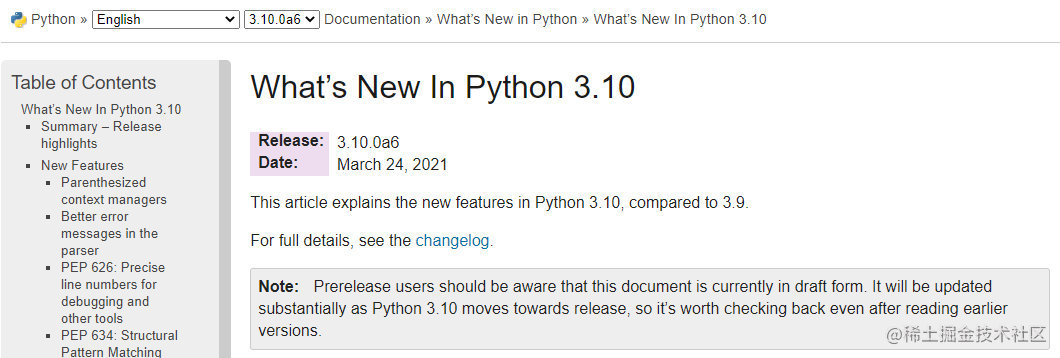# 最新总结：2021那些小众精巧的 Python 语法汇总！

2020 年 python2 停止维护,公司代码规范也鼓励使用 python3.6+版本,而随着 Python 版本的不断更新,许多旧的语法在可读性与效率上都已经有更好的替代了。当然,大部分的重要特性，例如装饰器、生成器、async 等，相信大家都非常熟悉了,这里就面向一些使用率稍微少一些、日常所见代码中不太常见的能用得上的语法做一个汇总，仅供参考。### PEP 3132 可迭代对象解包拓展

Python3.0 引入，加强了原本的星号运算符(*)，让星号运算符能够智能地展开可迭代对象。

``````>>> a, *b, c = range(5)
>>> a
0
>>> c
4
>>> b
[1, 2, 3]

``````>>> for a, *b in [(1, 2, 3), (4, 5, 6, 7)]:
>>>     print(b)
[2, 3]
[5, 6, 7]

### PEP 465 矩阵乘法运算符

Python3.5 引入，顾名思义，使用@符号。直接支持 numpy、pandas 等使用。

``````>>> a = numpy.array([1, 2, 3])
>>> b = numpy.array([10, 20, 30])
>>> a @ b
140

>>> c = numpy.array([[10, 15], [20, 25], [30, 35]])
>>> d = numpy.array([[4, 5, 6], [7, 8, 9]])
>>> c @ d
array([[145, 170, 195],
[255, 300, 345],
[365, 430, 495]])

``````>>> from math import sqrt

>>> class Point:
>>>     def __init__(self, x, y):
>>>         self.x = x
>>>         self.y = y
>>>
>>>     def __matmul__(self, value):
>>>         x_sub = self.x - value.x
>>>         y_sub = self.y - value.y
>>>         return sqrt(x_sub**2 + y_sub**2)
>>>
>>> a = Point(1, 3)
>>> b = Point(4, 7)
>>> print(a @ b)
5

### PEP 3107/484/526 函数注解/类型提示/变量注解

Python3.0 引入函数注解、3.5 引入 typing，让 python 也能享受静态类型的福利。可以说是 py3 中个人最喜欢的 feature，使用简单、效果强大，直接让开发效率以及代码可维护性直线增长。

``````# 参数后加:即可标注类型，函数结构定义后接->即可标注返回类型
def get_hello(name: str) -> str:
return f"Hello, {name}!"

www.python.org/dev/peps/pe…

``````from typing import TypeVar, Iterable, Tuple

T = TypeVar('T', int, float, complex)
Vector = Iterable[Tuple[T, T]]

def inproduct(v: Vector[T]) -> T:
return sum(x*y for x, y in v)

def dilate(v: Vector[T], scale: T) -> Vector[T]:
return ((x * scale, y * scale) for x, y in v)

vec = []  # type: Vector[float]

``````pi: float = 3.142

# 也同样支持Union等
from typing import Union

a: Union[float,None] =1.0

3.7 中又引入了延迟标记求值(PEP 563)，让 typing 支持了前向引用、并减轻了标注对程序启动时间的影响，如虎添翼。

``````# 3.7前合法
class Tree:
def __init__(self, left: 'Tree', right: 'Tree'):
self.left = left
self.right = right

# 3.7前不合法、3.7后合法
class Tree:
def __init__(self, left: Tree, right: Tree):
self.left = left
self.right = right

github.com/realpython/…

### PEP 498 f-string

Python3.6 引入，应该是用的最多的 feature 之一了，但是看到很多代码里面还是 str.format，就不得不再提一下。

``````>>> a = 10
>>> #只需要简单的在任意字符串字面量前加个f，就可以用花括号直接引用变量
>>> print(f"a = {a}")
a = 10

>>> # 格式化也很方便，使用:即可
>>> pi = 3.14159
>>> print(f"pi = {pi: .2f}")
pi = 3.14

``````>>> theta = 30

### PEP 515 数值字面值下划线

Python3.6 引入。输入太长的数字字面值怎么办？

``````>>> a = 123_456_789
>>> b = 123456789
>>> a == b
True

### PEP 557 数据类 Data Classes

``````from dataclasses import dataclass

@dataclass
class InventoryItem:
name: str
unit_price: float
quantity_on_hand: int = 0

def total_cost(self) -> float:
return self.unit_price * self.quantity_on_hand

``````def __init__(self, name: str, unit_price: float, quantity_on_hand: int = 0) -> None:
self.name = name
self.unit_price = unit_price
self.quantity_on_hand = quantity_on_hand
def __repr__(self):
return f'InventoryItem(name={self.name!r}, unit_price={self.unit_price!r}, quantity_on_hand={self.quantity_on_hand!r})'
def __eq__(self, other):
if other.__class__ is self.__class__:
return (self.name, self.unit_price, self.quantity_on_hand) == (other.name, other.unit_price, other.quantity_on_hand)
return NotImplemented
def __ne__(self, other):
if other.__class__ is self.__class__:
return (self.name, self.unit_price, self.quantity_on_hand) != (other.name, other.unit_price, other.quantity_on_hand)
return NotImplemented
def __lt__(self, other):
if other.__class__ is self.__class__:
return (self.name, self.unit_price, self.quantity_on_hand) < (other.name, other.unit_price, other.quantity_on_hand)
return NotImplemented
def __le__(self, other):
if other.__class__ is self.__class__:
return (self.name, self.unit_price, self.quantity_on_hand) <= (other.name, other.unit_price, other.quantity_on_hand)
return NotImplemented
def __gt__(self, other):
if other.__class__ is self.__class__:
return (self.name, self.unit_price, self.quantity_on_hand) > (other.name, other.unit_price, other.quantity_on_hand)
return NotImplemented
def __ge__(self, other):
if other.__class__ is self.__class__:
return (self.name, self.unit_price, self.quantity_on_hand) >= (other.name, other.unit_price, other.quantity_on_hand)
return NotImplemented

### PEP 572 海象牙运算符"逼走"了 Guido van Rossum，最有争议的 PEP 之一。首先引入了海象牙运算符`:=`，代表行内赋值。

``````# Before
while True:
command = input("> ");
if command == "quit":
break
print("You entered:", command)

# After
while (command := input("> ")) != "quit":
print("You entered:", command)

assignment expressions 在进行分支判断时非常好用，写的时候能够舒服很多。本身使用也集中在 if/while 这种场景，虽然让语法变复杂了，但是总体还是可控的，舒适程度大于风险。

``````temp_list = ["abc","bcd"]
result_list = (x for x in range(len(temp_list)))
print(list(result_list))

# 等价于
# Before
temp_list = ["abc", "bcd"]

def func_data(data: int):
for x in range(data):
yield x

result_list = func_data(len(temp_list))
print(list(result_list))

# After
temp_list = ["abc", "bcd"]

def func_data():
for x in range(len(temp_list)):
yield x

result_list = func_data()
print(list(result_list))

python 社区在激烈辩论后，这一部分的修改被成功撤销，只保留了海象牙运算符。

### PEP 570 仅限位置形参

``````def f(a, b, /, c, d, *, e, f):
print(a, b, c, d, e, f)

# 以下调用均合法
f(10, 20, 30, d=40, e=50, f=60)

# 以下调用均不合法
f(10, b=20, c=30, d=40, e=50, f=60)   # b cannot be a keyword argument
f(10, 20, 30, 40, 50, f=60)           # e must be a keyword argument

`/`语法的添加让调用函数时可以在可读性与简洁之间自由选择，可以选择强制不接受关键字参数、不需要形参名称时也可以省略。同时也让接受任意参数函数的实现变得方便了许多，例如：

``````class Counter(dict):
def __init__(self, iterable=None, /, **kwds):
# Note "iterable" is a possible keyword argument

### PEP 584 字典合并运算符

``````a={'a':1,'b':2}
b={'c':3}

a.update(b)

# 或者是
c = {**a, **b}

``````a |= b
c = a | b

### PEP 617 / bpo-12782 括号内的上下文管理

``````with (CtxManager() as example):
...

with (
CtxManager1(),
CtxManager2()
):
...

with (CtxManager1() as example,
CtxManager2()):
...

with (CtxManager1(),
CtxManager2() as example):
...

with (
CtxManager1() as example1,
CtxManager2() as example2
):
...

### PEP 634 结构化模式匹配 match-case

``````match subject:
case <pattern_1>:
<action_1>
case <pattern_2>:
<action_2>
case <pattern_3>:
<action_3>
case _:
<action_wildcard>

``````def http_error(status):
match status:
case 400:
case 401 | 403 | 404:
return "Not allowed"
case 404:
case 418:
return "I'm a teapot"
case _:
return "Something's wrong with the Internet"

``````# point is an (x, y) tuple
match point:
case (0, 0):
print("Origin")
case (0, y):
print(f"Y={y}")
case (x, 0):
print(f"X={x}")
case (x, y):
print(f"X={x}, Y={y}")
case _:
raise ValueError("Not a point")

### 结语

Python语言的发展是由技术方面的进步、工程方面的刚需汇聚而成的智慧结晶，身在其中便能体会到来自码农们创造出的代码设计之巧思、学问。只有尽可能多去理解各类语法意义，才能让开发变得流畅；了解语法的构成与其争议，在计算机科学领域的视野才会豁然开朗。与时俱进才是真正的好码神~这篇文章就到这里，如果对你有帮助的话不妨点赞、收藏、转发，欢迎在评论区交流以及提出宝贵意见，更多的Python实战技巧，学习资料欢迎私信与我交流，我会尽我所能的提供帮助!